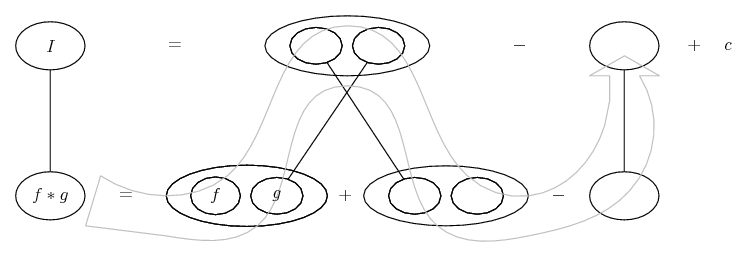# Thread: Very difficult integration.

1. ## Very difficult integration.

I'm really having difficulty integrating this, everytime I start I just ending up with workings that go on forever, and using Mathcad is just giving me useless answers.

Can anybody please give me some tips, rules, any help at all.

$\displaystyle \int[(1-2cosx)(3+2cosx)e^{\frac{1}{2}x+cosx}]dx$

Many thanks.

2. ## Re: Very difficult integration.

Can I apply The Constant Multiple Rule to

$\displaystyle e^{\frac{1}{2}x+cosx}$

and put it outside of the problem, then integrate the remaining parts?

3. ## Re: Very difficult integration.Originally Posted by SrengamCan I apply The Constant Multiple Rule to

$\displaystyle e^{\frac{1}{2}x+cosx}$

and put it outside of the problem, then interate the remaining parts?
no, you can't.

fyi, wolfram alpha says the antiderivative is $\displaystyle -2(1+2\sin{x})e^{x/2 + \cos{x}} + C$ ... won't show the steps, though.

I'm pretty sure it's some clever substitution or trig identity manipulation that I can't see

4. ## Re: Very difficult integration.

That actually makes a bit more sense to me now to be honest, it's similar to a previous part of the question. I think I might not have to show all the workings, just how to get to a derivative from a previous question, and then say (and since we know from the previous question . . .).

Many thanks Skeeter, I'll continue trying to manipulate things to get there.

5. ## Re: Very difficult integration.

... it's similar to a previous part of the question.
just so you know, it helps one to see the entire problem when trying to provide assistance.

6. ## Re: Very difficult integration.

It's indeed a very tricky one, I also don't see any clue to solve this integral.

7. ## Re: Very difficult integration.

Sorry,

I didn't want to ask for too much though, I figured if somebody could just give me a clue or a hint on how to integrate it, I would be OK.

I don't like asking for too much, it's a bit unfair on you.

8. ## Re: Very difficult integration.

No, not at all. You don't ask too much. I'm also very curious to a solution for this integral. I tried several things, but none of them seems to work.

9. ## Re: Very difficult integration.

One way to integrate involves solving a first-order linear ordinary differential equation. First, note that

$\displaystyle \left(\frac{u}{v}\right)'=\frac{vu'-uv'}{v^{2}}.$

That, of course, is just the quotient rule for derivatives. You can integrate it once to obtain

$\displaystyle \frac{u}{v}+C=\int\frac{vu'-uv'}{v^{2}}\,dx.$

Now, if you could get the integrand to look like the integrand I just mentioned, you'd be done. Let's say you write

$\displaystyle \int\frac{e^{-x/2-\cos(x)}(1-2\cos(x))(3+2\cos(x))}{e^{-x-2\cos(x)}}\,dx.\quad(1)$

All I've done is write the exponential in the denominator, and then multiplied top and bottom by the new denominator, because I want to get a $\displaystyle v^{2}$ in the denominator. So now I want

$\displaystyle v=e^{-x/2-\cos(x)}.$ This forces my quotient rule to look like this:

$\displaystyle \frac{vu'-uv'}{v^{2}}=\frac{e^{-x/2-\cos(x)}u'-e^{-x/2-\cos(x)}(-1/2+\sin(x))u}{e^{-x-2\cos(x)}}}.$

Equating the numerator of this RHS with the previous numerator of (1) yields the first-order linear ordinary differential equation

$\displaystyle u'-(-1/2+\sin(x))u=(1-2\cos(x))(3+2\cos(x)).$

The solution to this DE is

$\displaystyle u=e^{-x/2-\cos(x)}C-2(1+2\sin(x)).$

Hence, the integration result is

$\displaystyle \frac{u}{v}=\frac{e^{-x/2-\cos(x)}C-2(1+2\sin(x))}{e^{-x/2-\cos(x)}}=C-2e^{x/2+\cos(x)}(1+2\sin(x)),$

as WolframAlpha yields.

10. ## Re: Very difficult integration.

Or, just pummel (1 - 2 cos x)(3 + 2 cos x) untill you can see the derivative of 1/2 x + cos x.

Multiply out...

$\displaystyle =\ 3 - 4 \cos x - 4 \cos^2 x$

Get sin squared from cos squared...

$\displaystyle =\ 4 \sin^2 x - 4 \cos x - 1$

Difference of two squares...

$\displaystyle =\ (2 \sin x + 1)(2 \sin x - 1) - 4 \cos x$

There's our derivative...

$\displaystyle =\ (\frac{1}{2} - \sin x)(-4 - 4 \sin x) - 4 \cos x$

So we can try and integrate

$\displaystyle e^{\frac{1}{2}x + \cos x} (\frac{1}{2} - \sin x)(-4 - 4 \sin x)$

by parts. We might even see that the other integrand...

$\displaystyle e^{\frac{1}{2}x + \cos x} (-4 \cos x)$

... is going to come in handy soon enough. In other words, it's a good example of problems that will yield to integration by parts but dazzle just because they contain both lower forks of the product rule...... instead of just one. (Key in spoiler...)

Spoiler:... is the product rule for differentiation. Straight lines differentiate downwards with respect to x.... is lazy integration by parts, doing without u and v. But into this we are going to embed...... the chain rule. Straight continuous lines again differentiate downwards (integrate up) with respect to the main variable (in this case x), and the straight dashed line similarly but with respect to the dashed balloon expression (the inner function of the composite which is subject to the chain rule).

Just in case a picture helps with the parts..... or else, zooming in on the chain rule...__________________________________________________ __________

Don't integrate - balloontegrate!

Balloon Calculus; standard integrals, derivatives and methods

Balloon Calculus Drawing with LaTeX and Asymptote!

,

,

### very difficult integral

Click on a term to search for related topics.

#### Search Tags

difficult, integration